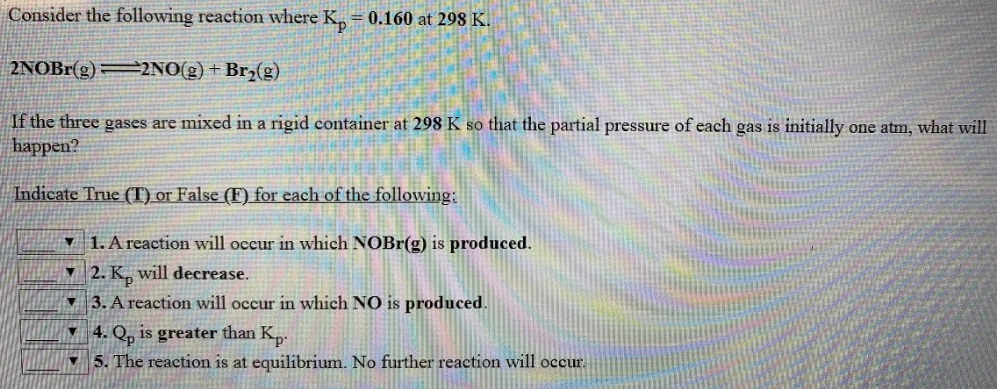# Consider the following reaction where Kp = 0.160 at 298 K. 2NOBr(g) ⇌ 2NO(g) + Br2(g) If the three gases are mixed in a rigid container at 298 K so that the partial pressure of each gas is initially one atm, what will happen? Indicate True (T) or False (F) for each of the following; 1. A reaction will occur in which NOBr(g) is produced. 2. Kp will decrease 3. A reaction will occur in which NO is produced. 4. Qp is greater than Kp. 5. The reaction is at equilibrium. No further reaction will occur.